Physical Chemistry : Atomic Nucleus

Example Questions

Example Question #31 : Nuclear, Quantum, And Molecular Chemistry

How many neutrons are present in the nucleus of one atom of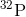Explanation:

From the periodic table, we find that the atomic number, i.e., the number of protons in the nucleus of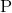is 15. Given that the mass number is 32, and recalling the formula which relates mass number to the number of neutrons and protons: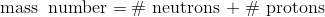, we find that the number of neutrons in the nucleus of one atom ofis 17.

Example Question #32 : Nuclear, Quantum, And Molecular Chemistry

Which atomic symbol represents a period five transition metal that has 42 electrons when it forms a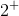cation?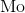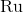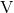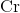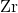Explanation:

Transition metals extend from groups 3 through 12 and periods 4 through 7. Ru, in its neutral state, has 44 electrons. Therefore, when it becomes acation, it will have 42 electrons.

Example Question #33 : Nuclear, Quantum, And Molecular Chemistry

What is the mass number, atomic number, and charge, of the isotope of an atom that contains 34 protons, 36 neutrons, and 36 electrons?

Mass number:Atomic number:Charge: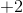Mass number:Atomic number:Charge:Mass number:Atomic number:Charge:Mass number:Atomic number:Charge:Mass number:Atomic number:Charge:Mass number:Atomic number:Charge:Explanation:

The atomic number is equal to the number of protons in the element, so from the periodic table, we find that the element with atomic number 34 is selenium. Since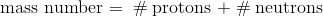, we calculate that the mass number is 70. Lastly, there are two more electrons than protons, so the charge will be.

All Physical Chemistry Resources